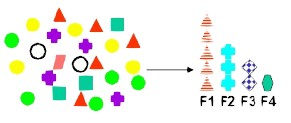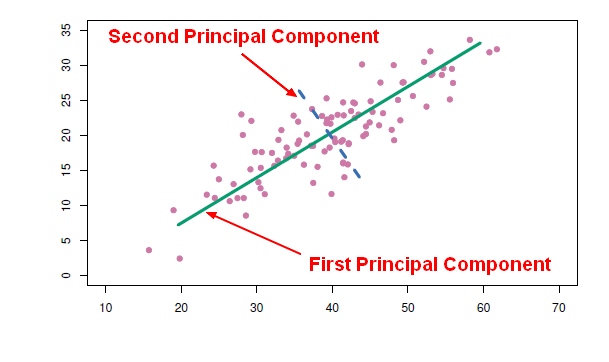# Data Mining - Partial least squares (PLS)

Partial least squares (PLS) is is a dimension reduction method and uses the same method than principle components regression but it selects the new predictors (principal component) in a supervised way.

The PLS approach attempts to find directions (ie principal component) that help explain both:

• the response
• and the original predictors.

PLS look for a direction in which the original predictors varies that are also related to the response.

## Steps

• The first partial least squares direction z1, is proportional to the correlation between the response y and the data matrix x.
• Subsequent directions are found by taking residuals and then repeating the above prescription.

## PLS vs PCR

In principle, partial least squares should be a huge gain over principle components regression because it chooses the direction looking at the response but in practice, PLS often does not give a huge gain over principle components regression (PCR).

Ridge and principle components regression work as well and are both simpler.

Recommended PagesData Mining - (Feature|Attribute) Extraction Function

Feature extraction is the second class of methods for dimension reduction. dimension reduction It creates new attributes (features) using linear combinations of the (original|existing) attributes. ...Data Mining - Principal Component (Analysis|Regression) (PCA|PCR)

Principal Component Analysis (PCA) is a feature extraction method that use orthogonal linear projections to capture the underlying variance of the data. By far, the most famous dimension reduction approach...